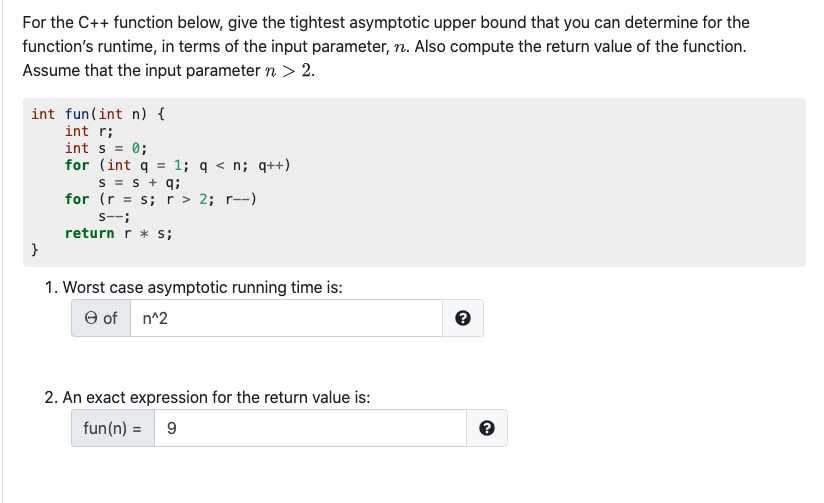# Question For the C++ function below, give the tightest asymptotic upper bound that you can determine for the function's runtime, in terms of the input parameter, n. Also compute the return value of the function. Assume that the input parameter n > 2. int fun(int n) { int r; int s = 0; for (int q = 1; q < n; (++) S = S + q; for (r = s; r > 2; r--) S--; return r * s; } 1. Worst case asymptotic running time is: of n^2 2 2. An exact expression for the return value is: fun(n) = 9AH9L2K The Asker · Computer ScienceTranscribed Image Text: For the C++ function below, give the tightest asymptotic upper bound that you can determine for the function's runtime, in terms of the input parameter, n. Also compute the return value of the function. Assume that the input parameter n > 2. int fun(int n) { int r; int s = 0; for (int q = 1; q < n; (++) S = S + q; for (r = s; r > 2; r--) S--; return r * s; } 1. Worst case asymptotic running time is: of n^2 2 2. An exact expression for the return value is: fun(n) = 9
More
Transcribed Image Text: For the C++ function below, give the tightest asymptotic upper bound that you can determine for the function's runtime, in terms of the input parameter, n. Also compute the return value of the function. Assume that the input parameter n > 2. int fun(int n) { int r; int s = 0; for (int q = 1; q < n; (++) S = S + q; for (r = s; r > 2; r--) S--; return r * s; } 1. Worst case asymptotic running time is: of n^2 2 2. An exact expression for the return value is: fun(n) = 9